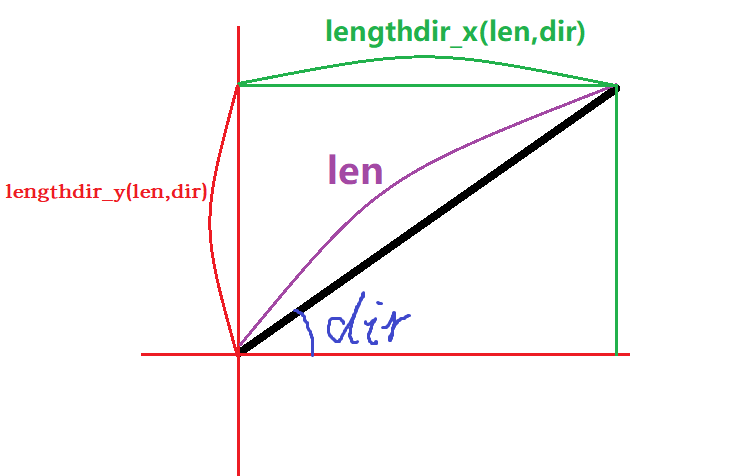GM8 可以使用许多的数学函数，如：

• `abs(x)` 返回 x 的绝对值
• `sign(x)` 返回 x 的符号（正数为 1，0 为 0，负数为 -1）
• `round(x)` 返回 x 的四舍五入
• `floor(x)` 返回比 x 小的最大整数
• `ceil(x)` 返回比 x 大的最小整数
• `frac(x)` 返回 x 的小数部分
• `sqrt(x)` 返回 x 的开方
• `sqr(x)` 返回 x 的平方
• `power(x, n)` 返回 x 的 n 次方
• `exp(x)` 返回 e 的 x 次方
• `ln(x)` 返回 x 的自然对数
• `log2(x)` 返回 x 关于 2 的对数
• `log10(x)` 返回 x 关于 10 的对数
• `logn(n, x)` 返回 x 关于 n 的对数
• `sin(x)` 返回 x 的正弦，x 为弧度制
• `cos(x)` 返回 x 的余弦，x 为弧度制
• `tan(x)` 返回 x 的正切，x 为弧度制
• `arcsin(x)` 返回 x 的反正弦
• `arccos(x)` 返回 x 的反余弦
• `arctan(x)` 返回 x 的反正切
• `arctan2(y, x)` 返回 y/x 的反正切，并且根据 y 和 x 自动纠正象限
• `degtorad(x)` 将角度 x 转换为弧度
• `radtodeg(x)` 将弧度 x 转换为角度
• `min(x1, x2, x3, ...)` 返回一系列数值中的最小值
• `max(x1, x2, x3, ...)` 返回一系列数值中的最大值
• `mean(x1, x2, x3, ...)` 返回一系列数值中的平均值
• `median(x1, x2, x3, ...)` 返回一系列数值中的中位数

• `random(x)` 返回一个 [0, x) 之间的随机实数（包含整数和小数）。
• `irandom(x)` 返回一个 [0, x] 之间的随机整数。
• `random_range(x1,x2)` 返回一个 [x1, x2) 之间的随机实数。
• `irandom_range(x1,x2)` 返回一个 [x1, x2] 之间的随机整数。
• `choose(val1,val2,val3,....)` 随机返回最多 16 个参数中的一个。
• `random_set_seed(seed)` 设置随机种子，随机种子要求是整数。
• `random_get_seed()` 返回当前正在使用的随机种子。
• `randomize()` 设置随机数作为随机种子。

### 随机数和随机种子

1. 所有三角函数均使用弧度制。其他的函数大都使用的是角度制。
2. 在 GM8 里，向右为 0°，向上为 90°，向左为 180°，向下为 270°，在使用角度时应多加注意。
3. GM8 的房间以左上角为原点，向右为 x 轴正方向，向下为 y 轴正方向。
• `lengthdir_x(len,dir)` 返回指定长度和角度的向量在 x 轴上的投影长度。使用角度制。
• `lengthdir_y(len,dir)` 返回指定长度和角度的向量在 y 轴上的投影长度。使用角度制。• `point_distance(x1, y1, x2, y2)` 返回坐标 (x1, y1) 与 (x2, y2) 的距离。
• `point_direction(x1, y1, x2, y2)` 返回坐标 (x2, y2) 相对于 (x1, y1) 的角度。
• `is_real(x)` 返回 x 是否为实数值。（返回 1 为实数值，返回 0 为字符串）
• `is_string(x)` 返回 x 是否为字符串。（返回 1 为字符串，返回 0 为实数值）